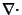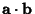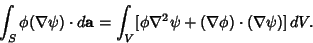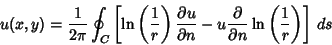## Green's Identities

Green's identities are a set of three vector derivative/integral identities which can be derived starting with the vector derivative identities(1)

and(2)

whereis the Divergence,is the Gradient,is the Laplacian, andis the Dot Product. From the Divergence Theorem,(3)

Plugging (2) into (3),(4)

This is Green's first identity.

Subtracting (2) from (1),(5)

Therefore,(6)

This is Green's second identity.

Lethave continuous first Partial Derivatives and be Harmonic inside the region of integration. Then Green's third identity is(7)

(Kaplan 1991, p. 361).

References